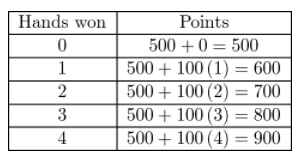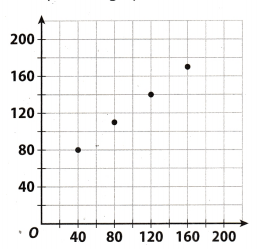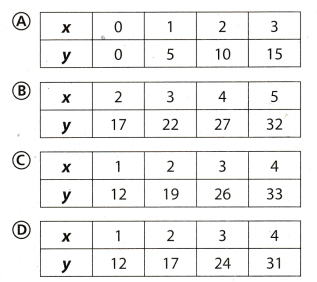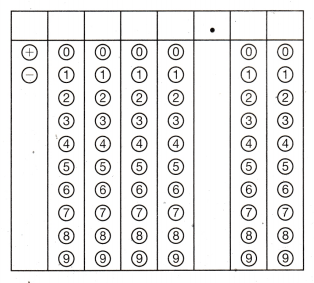Refer to our Texas Go Math Grade 7 Answer Key Pdf to score good marks in the exams. Test yourself by practicing the problems from Texas Go Math Grade 7 Module 7 Quiz Answer Key.

7.1 Linear Relationships in the Form y = mx + b

Question 1.
Darice also took a break after riding 10 miles. The table below shows the rate at which Darice rides her bicycle after the break.Write a verbal description of the relationship between the time she rides and the distance she travels.
Dance was riding a bike 10 miles plus an additional 0.25 miles after each break.

7.2 Writing and Graphing Equations in the Form y = mx + b

Emir started out a card game with 500 points. For every hand he won, he gained 100 points.
Question 2.
Complete the table.y = 100 ∙ x + 500

Question 3.
Plot the points on the graph.y = 100 ∙ x + 500

Question 4.
Write an equation for the linear relationship.
y = 100 ∙ x + 500

Essential Question

Question 5.
What are some of the ways you can represent real-world linear relationships?
Real-world relationships can be represented by graphics. tables, and equations.

Texas Go Math Grade 7 Module 7 Mixed Review Texas Test Prep Answer Key

Selected Response

Question 1.
Which description corresponds to the relationship shown in the table?(A) earning $10 an hour (B) earning$8 an hour plus a $10 bonus (C) earning$7 an hour plus a $15 bonus (D) earning$9 an hour
(B) earning $8 an hour plus a$10 bonus

Explanation:
90 – 50 = 40 Find the diferrence between two payments.
$$\frac{40}{5}$$ = 8 Find how much is paid for 1 hour.
50 – 40 = 10 Find a bonus.

Question 2.
Which equation represents the same linear relationship as the graph below?(A) y = 1.2x + 32
(B) y = 1.5x + 20
(C) y = 0.75x + 50
(D) y = 0.8x + 45
(C) y = 0.75 ∙ x + 50

Explanation:
Our points on the graph are:
A = (40,80)
B = (80,110)
C = (120, 140)
D = (160, 170)
Replacing the value of point A in the solution under (A) we get:
80 = 1.2 ∙ 40 + 32 = 80
This is correct
Replacing the value of point B in the solution under (A) we get:
110 = 1.2 ∙ 80 + 32 = 128
This isn’t correct.

By repeating the procedure, we will conclude that alt points are solutions of the equation under (C)

Question 3.
Omar began the week with $25. He took a city bus to and from school, paying$1.25 for each trip. Let x be the number of trips he took and y be the amount of money he had left at the end of the week. Which equation represents the relationship in the situation?
(A) y = 1.25x + 25
(B) y = 25 – 1.25x
(C) x = 25 – 1.25y
(D) y = 1.25x – 25
(B) y = 25 – 1.25x

Explanation:
The right answer is (B) y = 25 – 1.25 ∙ x, because he spends money on the bus, so the sum of money that remains to be reduced after each payment of trip.

Question 4.
Which table represents the same linear relationship as the equation y = 5x + 7?Table (B)

Explanation:
The table represent the equation
y = 5 ∙ x + 7

Question 5.
Selina is planning to paint a large picture on a wall. She draws a smaller version first. The drawing is 8 inches by 6 inches. If the scale of the drawing is 2 in: 1 ft, what is the area of the actual picture on the wall?
(A) 4 feet
(B) 3 feet
(C) 48 square inches
(D) 12 square feet
(D) 12 square feet

Explanation:
The answer is (D) 12 square feet
The area of the drawing is 8 ∙ 6 = 48 in.
Because scale of the drawing, the area of the actual picture on the wall is
$$\frac{8}{2} \cdot \frac{6}{2}$$ = 4 ∙ 3 = 12 square feet

Gridded Response

Question 6.
The equation y = 3.5x – 210 represents the profit made by a manufacturer that sells products for \$3.50 each, where y is the profit and x is the number of units sold. What is the profit in dollars when 80 units are sold?